# Write the logarithm as a sum or difference of logarithms. Simplify each term as much as...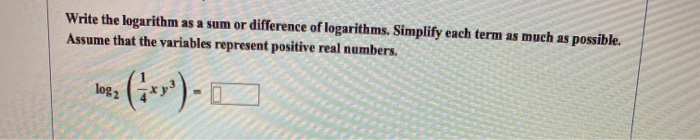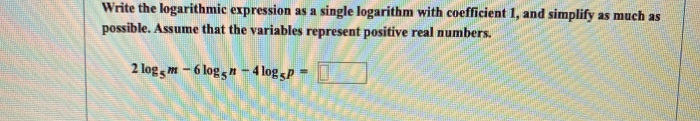Write the logarithm as a sum or difference of logarithms. Simplify each term as much as possible. Assume that the variables represent positive real numbers. log2 (**»*)- 0
Write the logarithm as a sum or difference of logarithms. Simplify each term as much as possible. Assume that the variables represent positive real numbers. mn In 3 Pa
Write the logarithmic expression as a single logarithm with coefficient 1, and simplify as much as possible. Assume that the variables represent positive real numbers. 2 log, m - 6 logg? - 4 loggp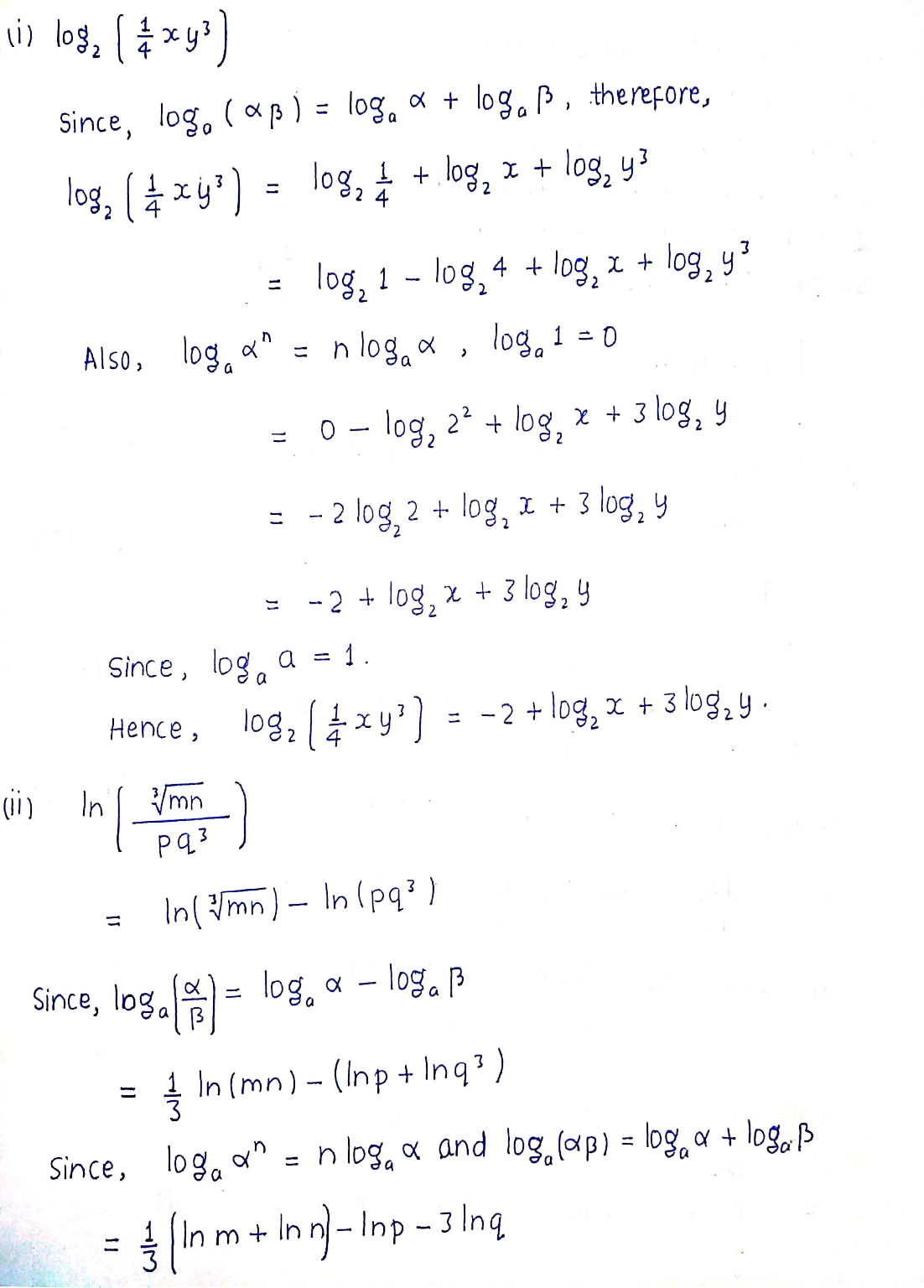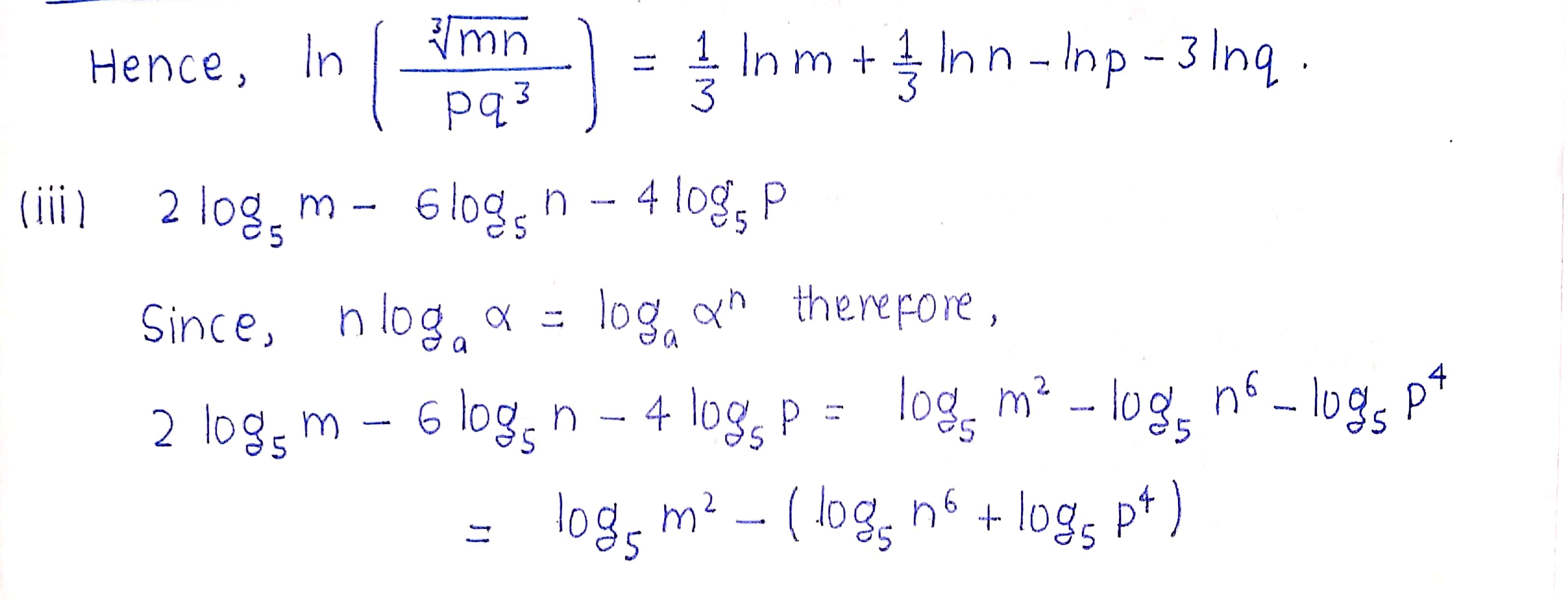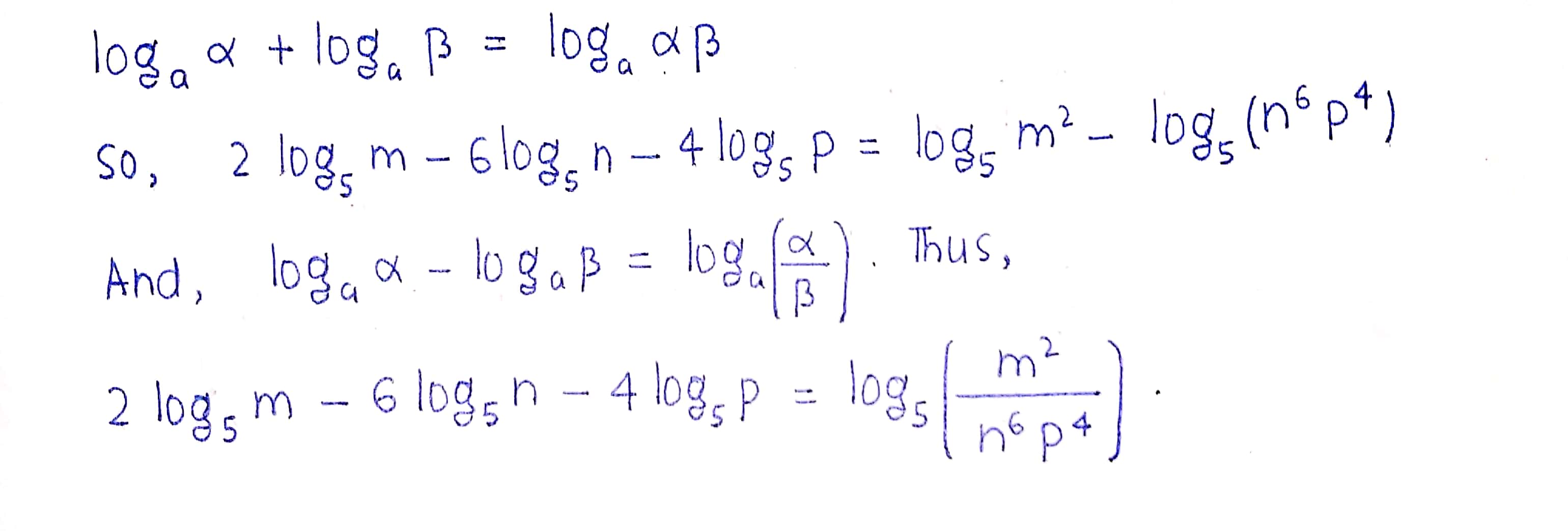##### Add Answer of: Write the logarithm as a sum or difference of logarithms. Simplify each term as much as...
Similar Homework Help Questions
• ### Write the logarithm as a sum or difference of logarithms. Simplify each term as much as...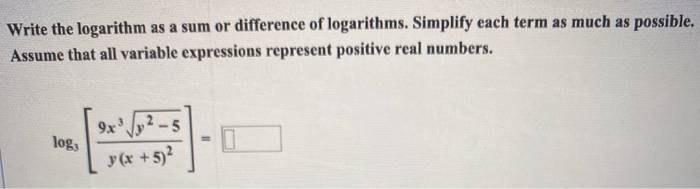Write the logarithm as a sum or difference of logarithms. Simplify each term as much as possible. Assume that all variable expressions represent positive real numbers. 9x/92-5 log y (x + 5)2

• ### Write the equation in exponential form. 1 log =-2 100 The equation in exponential form is...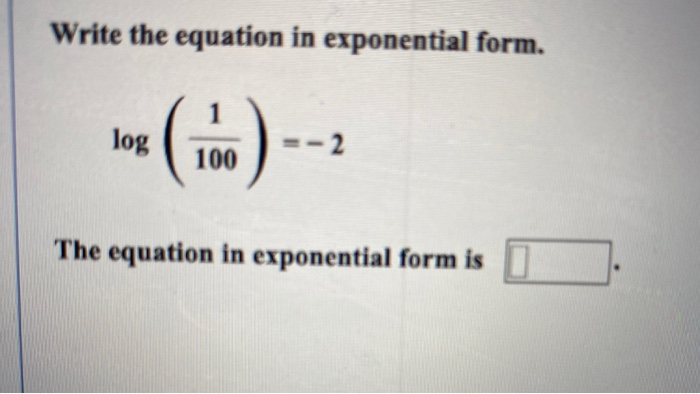Write the equation in exponential form. 1 log =-2 100 The equation in exponential form is Write the equation in logarithmic form. 10º = 1,000,000,000 The equation in logarithmic form is Simplify the expression. log 0.0001 = Write the logarithm as a sum or difference of logarithms. Simplify each term as much as possibl Assume that the variables represent positive real numbers. Vab In Write the logarithm as a sum or difference of logarithms. Simplify each term as much as...

• ### Use log, 3 * 0.583, log, 5 0.821, and log, 7. 1.064 to approximate the value...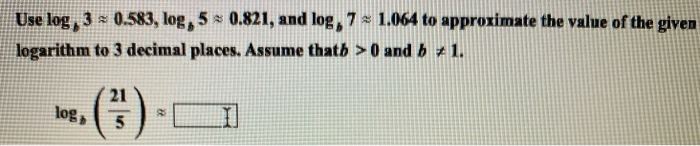Use log, 3 * 0.583, log, 5 0.821, and log, 7. 1.064 to approximate the value of the given logarithm to 3 decimal places. Assume thath > 0 and b + 1. log) 21 5. Write the logarithmic expression as a single logarithm with coefficient 1, and simplify as much possible. Assume that all variable expressions represent positive real numbers. log, (v) + log2 (x2 - 9) – log2 ( +3) - Write the logarithmic expression as a single logarithm...

• ### Write the logarithm as a sum or difference of logarithms. Simplify each term as much as...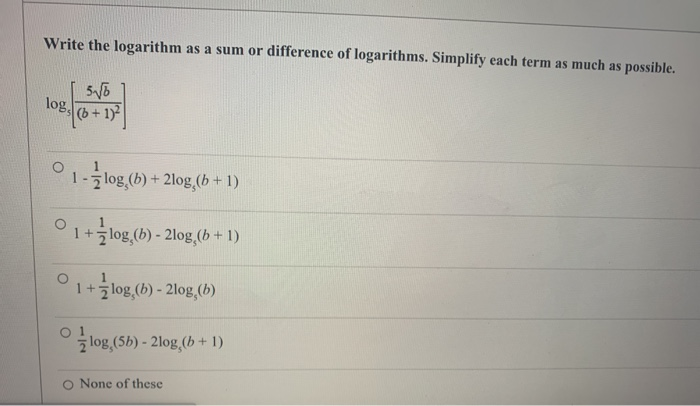Write the logarithm as a sum or difference of logarithms. Simplify each term as much as possible. 5V6 log, (6 + 1)2 1-3 log,(6) + 2log,(b + 1) 0 1 +žlog, (b) - 2log,66 + 1) 1 + Źlog (b) - 2log (b) îlog,(5b) - 2log, (b + 1) O None of these

• ### Write the logarithmic expression as a single logarithm with coefficient 1, and simplify as much as...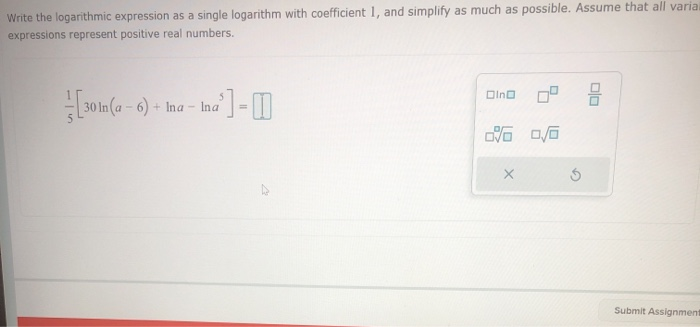Write the logarithmic expression as a single logarithm with coefficient 1, and simplify as much as possible. Assume that all varia expressions represent positive real numbers. Dino 3 [30.1 (a – 6) + Ina – Ina % 0/0 X Submit Assignment

• ### Previous 21 22 23 Next Question 21 of 23 (1 point) Apply the power property of...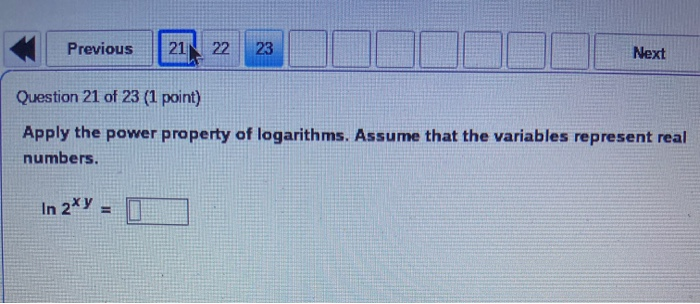Previous 21 22 23 Next Question 21 of 23 (1 point) Apply the power property of logarithms. Assume that the variables represent real numbers. In 2y = Question 22 of 23 (1 point) Write the logarithm as a sum or difference of logarithms. Simplify each term as much as possible. Assume that the variables represent positive real numbers. log3

• ### Use properties of logarithms to condense the logarithmic expression. Write the expression as a...

Use properties of logarithms to condense the logarithmic expression. Write the expression as a single logarithm whose coefficient is 1. where possible, evaluatelogarithmic expressions.log2+log5 =

• ### Need help with Algebra and Trigonometry questions.

graph each function f(x)= log 10 xGraph each function f(x)= 4xUse the properties of logarithms to rewrite each expression. simplify the result if possible. assume all variables represent positive real numbers.log2 2/3 / 5Given log 10 2= .3010 and log 10 3= .4771, Find each logarithm without using a calculator. log 10 3/2

• ### 2 1 points My No Rewrite the logarithmic expression as the sum and difference of logarithms. If exponents may be wr...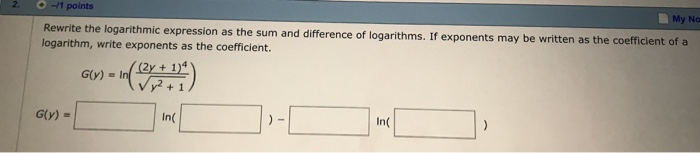2 1 points My No Rewrite the logarithmic expression as the sum and difference of logarithms. If exponents may be written as the coefficient of a logarithm, write exponents as the coefficient. (2y+ 1)4 Vy2 G(y) In +1 G(y)= In In 2 1 points My No Rewrite the logarithmic expression as the sum and difference of logarithms. If exponents may be written as the coefficient of a logarithm, write exponents as the coefficient. (2y+ 1)4 Vy2 G(y) In +1 G(y)=...

• ### The intensities of earthquakes are measured with seismographs all over the world at different distances from...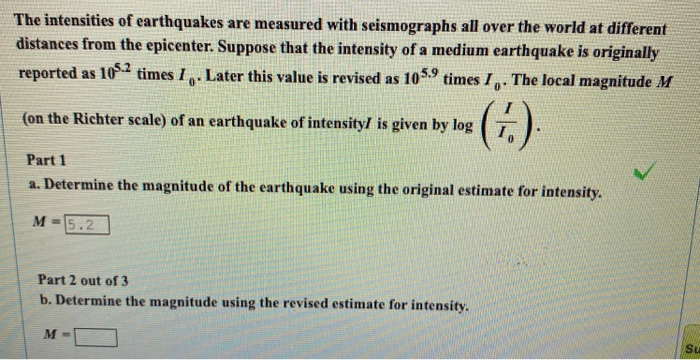The intensities of earthquakes are measured with seismographs all over the world at different distances from the epicenter. Suppose that the intensity of a medium earthquake is originally reported as 105.2 times 1. Later this value is revised as 105.9 times I. The local magnitude M (on the Richter scale) of an earthquake of intensity is given by log (6) Part 1 a. Determine the magnitude of the earthquake using the original estimate for intensity. M = 5.2 Part 2...

Free Homework App